### electronic universe

Nothing possible without electronics

.

## Norton's Theorem

In some ways Norton's Theorem can be thought of as the opposite to "Thevenins Theorem", in that Thevenin reduces his circuit down to a single resistance in series with a single voltage. Norton on the other hand reduces his circuit down to a single resistance in parallel with a constant current source. Norton's Theorem states that "Any linear circuit containing several energy sources and resistances can be replaced by a single Constant Current generator in parallel with a Single Resistor". As far as the load resistance, RL is concerned this single resistance, RS is the value of the resistance looking back into the network with all the current sources open circuited and IS is the short circuit current at the output terminals as shown below.

### Norton's equivalent circuit.

The value of this "Constant Current" is one which would flow if the two output terminals where shorted together while the source resistance would be measured looking back into the terminals, (the same as Thevenin).

For example, consider our now familiar circuit from the previous section.

To find the Nortons equivalent of the above circuit we firstly have to remove the centre 40Ω load resistor and short out the terminals A and B to give us the following circuit.

When the terminals A and B are shorted together the two resistors are connected in parallel across their two respective voltage sources and the currents flowing through each resistor as well as the total short circuit current can now be calculated as:

### with A-B Shorted Out

If we short-out the two voltage sources and open circuit terminals A and B, the two resistors are now effectively connected together in parallel. The value of the internal resistor Rs is found by calculating the total resistance at the terminals A and B giving us the following circuit.

### Find the Equivalent Resistance (Rs)

Having found both the short circuit current, Is and equivalent internal resistance, Rs this then gives us the following Nortons equivalent circuit.

### Nortons equivalent circuit.

Ok, so far so good, but we now have to solve with the original 40Ω load resistor connected across terminals A and B as shown below.

Again, the two resistors are connected in parallel across the terminals A and B which gives us a total resistance of:

The voltage across the terminals A and B with the load resistor connected is given as:

Then the current flowing in the 40Ω load resistor can be found as:

which again, is the same value of 0.286 amps, we found using Kirchoff's circuit law in the previous tutorials.

## Nortons Analysis Summary.

The basic procedure for solving Nortons Analysis equations is as follows:

• 1. Remove the load resistor RL or component concerned.
• 2. Find RS by shorting all voltage sources or by open circuiting all the current sources.
• 3. Find IS by placing a shorting link on the output terminals A and B.
• 4. Find the current flowing through the load resistor RL.

### Thevenins Theorem

Thevenins Theorem states that "Any linear circuit containing several voltages and resistances can be replaced by just a Single Voltage in series with a Single Resistor". In other words, it is possible to simplify any "Linear" circuit, no matter how complex, to an equivalent circuit with just a single voltage source in series with a resistance connected to a load as shown below. Thevenins Theorem is especially useful in analyzing power or battery systems and other interconnected circuits where it will have an effect on the adjoining part of the circuit.

### Thevenins equivalent circuit.As far as the load resistor RL is concerned, any "One-port" network consisting of resistive circuit elements and energy sources can be replaced by one single equivalent resistance Rs and voltage Vs, where Rs is the source resistance value looking back into the circuit and Vs the open circuit voltage at the terminals.

For example, consider the circuit from the previous section.Firstly, we have to remove the centre 40Ω resistor and short out (not physically as this would be dangerous) all the emf´s connected to the circuit, or open circuit any current sources. The value of resistor Rs is found by calculating the total resistance at the terminals A and B with all the emf´s removed, and the value of the voltage required Vs is the total voltage across terminals A and B with an open circuit and no load resistor Rs connected. Then, we get the following circuit.### Find the Equivalent Resistance (Rs)### Find the Equivalent Voltage (Vs)We now need to reconnect the two voltages back into the circuit, and as VS = VAB the current flowing around the loop is calculated as:so the voltage drop across the 20Ω resistor can be calculated as:

VAB = 20 - (20Ω x 0.33amps) = 13.33 volts.

Then the Thevenins Equivalent circuit is shown below with the 40Ω resistor connected.and from this the current flowing in the circuit is given as:which again, is the same value of 0.286 amps, we found using Kirchoff´s circuit law in the previous tutorial.

Thevenins theorem can be used as a circuit analysis method and is particularly useful if the load is to take a series of different values. It is not as powerful as Mesh or Nodal analysis in larger networks because the use of Mesh or Nodal analysis is usually necessary in any Thevenin exercise, so it might as well be used from the start. However, Thevenins equivalent circuits of Transistors, Voltage Sources such as batteries etc, are very useful in circuit design.

## Summary.

The basic procedure for solving Thevenins Analysis equations is as follows:

• 1. Remove the load resistor RL or component concerned.
• 2. Find RS by shorting all voltage sources or by open circuiting all the current sources.
• 3. Find VS by the usual circuit analysis methods.
• 4. Find the current flowing through the load resistor RL.

## September 02, 2009

### SR Flip Flop

An SR Flip-Flop can be considered as a basic one-bit memory device that has two inputs, one which will "SET" the device and another which will "RESET" the device back to its original state and an output Q that will be either at a logic level "1" or logic "0" depending upon this Set/Reset condition. A basic NAND Gate SR flip flop circuit provides feedback from its outputs to its inputs and is commonly used in memory circuits to store data bits. The term "Flip-flop" relates to the actual operation of the device, as it can be "Flipped" into one logic state or "Flopped" back into another.

The simplest way to make any basic one-bit Set/Reset SR flip-flop is to connect together a pair of cross-coupled 2-input NAND Gates to form a Set-Reset Bistable or a SR NAND Gate Latch, so that there is feedback from each output to one of the other NAND Gate inputs. This device consists of two inputs, one called the Reset, R and the other called the Set, S with two corresponding outputs Q and its inverse or complement Q.

SR Flip Flop Component OutlineSR Flip Flop Circuit Diagram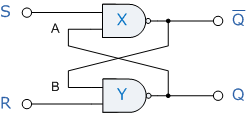### "Set" State

Consider the circuit shown above. If the input R is at logic level "0" (R = 0) and input S is at logic level "1" (S = 1), the NAND Gate Y has at least one of its inputs at logic "0" therefore, its output Q must be at a logic level "1" (NAND Gate principles). Output Q is also fed back to input A and so both inputs to the NAND Gate X are at logic level "1", and therefore its output Q must be at logic level "0". Again NAND gate principals. If the Reset input R changes state, and now becomes logic "1" with S remaining HIGH at logic level "1", NAND Gate Y inputs are now R = "1" and B = "0" and since one of its inputs is still at logic level "0" the output at Q remains at logic level "1" and the circuit is said to be "Latched" or "Set" with Q = "1" and Q = "0".

### "Reset" State

In this second stable state, Q is at logic level "0", Q = "0" its inverse output Q is at logic level "1", not Q = "1", and is given by R = "1" and S = "0". As gate X has one of its inputs at logic "0" its output Q must equal logic level "1" (again NAND gate principles). Output Q is fed back to input B, so both inputs to NAND gate Y are at logic "1", therefore, Q = "0". If the set input, S now changes state to logic "1" with R remaining at logic "1", output Q still remains LOW at logic level "0" and the circuit's "Reset" state has been latched.

### Truth Table for this Set-Reset Function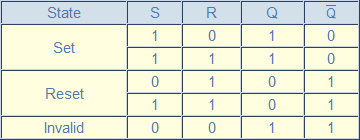It can be seen that when both inputs S = "1" and R = "1" the outputs Q and Q can be at either logic level "1" or "0", depending upon the state of inputs S or R BEFORE this input condition existed. However, input state R = "0" and S = "0" is an undesirable or invalid condition and must be avoided because this will give both outputs Q and Q to be at logic level "1" at the same time and we would normally want Q to be the inverse of Q. However, if the two inputs are now switched HIGH again after this condition to logic "1", both the outputs will go LOW resulting in the flip-flop becoming unstable and switch to an unknown data state based upon the unbalance. This unbalance can cause one of the outputs to switch faster than the other resulting in the flip-flop switching to one state or the other which may not be the required state and data corruption will exist. This unstable condition is known as its Meta-stable state.

Then, a bistable latch is activated or Set by a logic "1" applied to its S input and deactivated or Reset by a logic "1" applied to its R. The SR Latch is said to be in an "invalid" condition (Meta-stable) if both the Set and Reset inputs are activated simultaneously.

As well as using NAND Gates, it is also possible to construct simple 1-bit SR Flip-flops using two NOR Gates connected the same configuration. The circuit will work in a similar way to the NAND gate circuit above, except that the invalid condition exists when both its inputs are at logic level "1" and this is shown below.

### NOR Gate SR Flip-flop## August 30, 2009

### Superposition Theorem

Superposition Theorem

Superposition theorem is one of those strokes of genius that takes a complex subject and simplifies it in a way that makes perfect sense.

The strategy used in the Superposition Theorem is to eliminate all but one source of power within a network at a time, using series/parallel analysis to determine voltage drops (and/or currents) within the modified network for each power source separately. Then, once voltage drops and/or currents have been determined for each power source working separately, the values are all “superimposed” on top of each other (added algebraically) to find the actual voltage drops/currents with all sources active.

Let’s look at our example circuit and apply Superposition Theorem to it: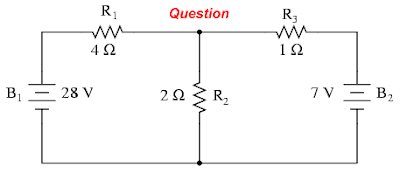Since we have two sources of power in this circuit, we will have to calculate two sets of values for voltage drops and/or currents, one for the circuit with only the 28 volt battery in effect. . .. . . and one for the circuit with only the 7 volt battery in effectWhen re-drawing the circuit for series/parallel analysis with one source, all other voltage sources are replaced by wires (shorts), and all current sources with open circuits (breaks).

Analyzing the circuit with only the 28 volt battery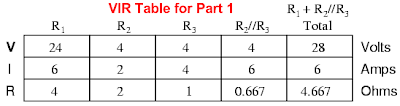Analyzing the circuit with only the 7 volt battery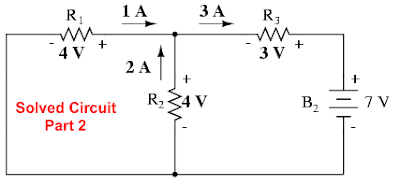Super Imposing VoltageSuper Imposing current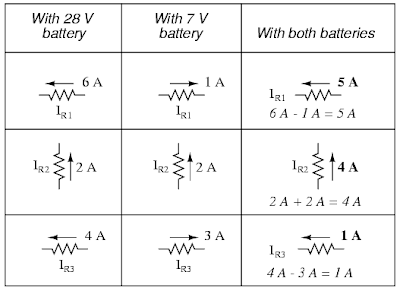Final CircuitREVIEW
The Superposition Theorem states that a circuit can be analyzed with only one source of power at a time, the corresponding component voltages and currents algebraically added to find out what they’ll do with all power sources in effect.

To negate all but one power source for analysis, replace any source of voltage (batteries) with a wire; replace any current source with an open (break).

DOOR BELL FOR DEAF CLICK HERE

Electronics is the study and use of electrical that operate by controlling the flow of electrons or other electrically charged particles in devices such as thermionic valves. and semiconductors. The pure study of such devices is considered as a branch of physics, while the design and construction electronic circuits to solve practical problems is called electronic engineering.